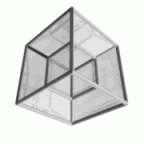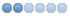If you want to share any helpful source code, libraries, tools, etc, to help programming with CT, this is the right place to do this.
 Page:1

# TOPIC:

## Raspberry PI and a little more 8 years 7 months ago #6750

• Javor
•Topic Author
• Offline
• Junior Member
•• M.Sc in Computer Science, (ex-)freelancer
• Posts: 72
Here's my 2 cents, used in my projects with Raspberry PI (currently tested only on model. I still don't have the new model B+, with the enhanced I/O or 2nd version of the board, so other people may confirm if it works with them. Anyway, here are my resources. First one is called rpi_hal.pas. It's NOT written by me, I've only made some slight modifications, because I had to talk to MCP23S17 expander and other SPI devices and currently functions didn't go for my purpose. All the information and the original rpi_hal.pas can be found/downloaded here . My unit is here:

#### File Attachment:

File Name: rpi_hal.zip
File Size:32 KB

Second thing is written by me and it's for LCD (16x2 up to 20x4 been tested), being connected to the MCP23S17 mentioned above. Code is extracted from a large unit (currently don't have time to separate it into a new one), with unnecessary LCD routines removed, so I hope I'll be excused this time. Here's the actual code:
```...
const
E_PULSE = 50; //usec
E_DELAY = 50; //usec

LCD_CHR = 1;
LCD_CMD = 0;

lcd_alignLeft   = 0;
lcd_alignCenter = 1;
lcd_alignRight  = 2;

LCD_LINE_1 = \$00; //\$80; // LCD RAM address for the 1st line
LCD_LINE_2 = \$40; //\$C0; // LCD RAM address for the 2nd line
LCD_LINE_3 = \$14; //\$94; // LCD RAM address for the 3rd line
LCD_LINE_4 = \$54; //\$D4; // LCD RAM address for the 4th line

lcd_init_array: array [0..3] of byte = (
\$28,  // Set mode: 4-bit, 2+ lines, 5x8 dots
\$0C,  // Display on
\$01,  // Clear display
\$06);

...
procedure pi_board_Write(addr: byte; reg: word; value: byte; const LowCS: Boolean = True; const HighCS: Boolean = True);
begin
if LowCS then
gpio_set_pin(gpio_pi_CS_23S17, output_Low); //gpio_pi_CS_23S17 is my chip-select PIN
//Sleep(50);
try
if LowCS then
SPI_Write_Byte(0, reg);
SPI_Write_Byte(0, value);
finally
if HighCS then
gpio_set_pin(gpio_pi_CS_23S17, output_High);
//Sleep(50);
end;
end;

procedure lcd_byte(bits: byte; mode: byte); //a low level function, see LCD_SendStringXY below
var data: byte;
begin
// Send byte to data pins
// bits = data
// mode = True  for character
//        False for command

data:=mode;
if LCD_BackLight_On then data:=data or \$08   // %0000 1000
else data:=data and \$F7; // %1111 0111
pi_board_Write(rpi_SPI_SLAVE_ADDR, GPIOB, data, True, True);//False); //RS

// High bits
//data:=0;
if bits and \$10 = \$10 then data:=data or \$10;
if bits and \$20 = \$20 then data:=data or \$20;
if bits and \$40 = \$40 then data:=data or \$40;
if bits and \$80 = \$80 then data:=data or \$80;

pi_board_Write(rpi_SPI_SLAVE_ADDR, GPIOB, data, True, True);//, False, False); //High bits

// toggle Enable bit
delay_us(E_DELAY);
data:=data or LCD_EN;
pi_board_Write(rpi_SPI_SLAVE_ADDR, GPIOB, data, True, True);//, False, False); //High bits
delay_us(E_PULSE);
data:=data and (255 - LCD_EN); //zero bit LCD_EN = 2
pi_board_Write(rpi_SPI_SLAVE_ADDR, GPIOB, data, True, True);//, False, False); //High bits
delay_us(E_DELAY);

// Low bits
data:=data and \$0F; //zero high 4 bits
if bits and \$1 = \$1 then data:=data or \$10;
if bits and \$2 = \$2 then data:=data or \$20;
if bits and \$4 = \$4 then data:=data or \$40;
if bits and \$8 = \$8 then data:=data or \$80;

pi_board_Write(rpi_SPI_SLAVE_ADDR, GPIOB, data, True, True);//, False, False); //High bits

// toggle Enable bit
delay_us(E_DELAY);
data:=data or LCD_EN;
pi_board_Write(rpi_SPI_SLAVE_ADDR, GPIOB, data, True, True);//, False, False); //High bits
delay_us(E_PULSE);
data:=data and (255 - LCD_EN); //zero bit LCD_EN = 2
pi_board_Write(rpi_SPI_SLAVE_ADDR, GPIOB, data, True, True);//, False, True); //High bits
delay_us(E_DELAY);
end;

procedure LCD_Init;
var I: Integer;
begin
lcd_byte(\$00, LCD_CMD);
for I:=0 to 2 do
begin
lcd_byte(\$03, LCD_CMD);
delay_msec(5);
end;
lcd_byte(\$02, LCD_CMD);
delay_msec(35);
for I:=0 to 3 do //currently 4-line display
begin
lcd_byte(LCD_Init_Array[I], LCD_CMD);
delay_msec(50);
end;
end;
procedure LCD_SendStringXY(X, Y: byte; what: ansistring; const align_: byte = lcd_alignLeft);
var b, c: byte;
I: Integer;
begin
b:=LCD_LINE_1;
case y of
2: b:=LCD_LINE_2;
3: b:=LCD_LINE_3;
4: b:=LCD_LINE_4;
end;

b:=b + x - 1;
lcd_byte(\$80 or b, LCD_CMD);

c:=Length(what);
if c > 20 then what:=Copy(what, 1, 20);

if c < 20 then
begin
if align_ = lcd_alignLeft then what:=what + StringOfChar(' ', 20 - c)
else
if align_ = lcd_alignRight then what:=StringOfChar(' ', 20 - c) + what
else
begin //align_ = lcd_alignCenter
if c mod 2 <> 0 then //odd count of string chars
begin
what:=what + ' ';
c:=Length(what);
end;
c:=(20 - c) div 2;
what:=StringOfChar(' ', c) + what + StringOfChar(' ', c);
end;
end;

for I:=1 to Length(what) do
begin
b:=Ord(What[I]);
lcd_byte(b, LCD_CHR);
end;
end;```
##### Attachments:
The following user(s) said Thank You: Stephen, George Christodoulou
 Page:1